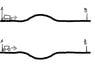# Slope/Hill velocity effect

• xtimmyx
In summary, the two wagons approaching the hill/slope will have the same velocity at point A. However, due to the conservation of energy, the lower wagon will have a higher velocity at point B while the first wagon will have a lower velocity. This is because the lower wagon will have increased kinetic energy while going through the slope, while the first wagon will have decreased kinetic energy. Eventually, they will both have the same velocity but the lower wagon will reach point B slightly sooner. This explains why, despite having the same starting velocity, the wagons will have different velocities at point B.

#### xtimmyx

Lets say two wagons is approaching a hill/slope which ends up at the exact same height as before the slope/hill. The wagons are identical and are traveling at the same velocity at point A (approaching the hill/slope). And the hill's/slope's shape is exactly the same but inverted.

Which if any, of the wagons reaches point B first, and which has the highest velocity at that point.

To me it seams logical that its the same for both wagons but as I remember it from a physics assignment in school a couple of years ago that isn't the case and the wagons time/velocity are different at point B.

If so, why?

#### Attachments

•slopeHillVelocity.jpg
11.9 KB · Views: 371
Looking at it in terms of "conservation of energy it is clear that the velocity of the two wagons will be the same at B- they are both at the same height they were before so have the same potential enter, thus the same kinetic energy and so the same velocity.

However, the lower wagon will have increased decreased potential energy, so increased kinetic energy and thus higher velocity while going through the while the first wagon has increased potential energy, so decreased kinetic energy and thus lower velocity while going over the hill. Afterwards, they will have the same velocity but the lower wagon will get to point B slightly sooner than the first wagon.

Thank you. It's nice too I still remember something at least. And that both that me and my colleague was "right" in a way.

## 1. What is the "Slope/Hill velocity effect"?

The "Slope/Hill velocity effect" refers to the phenomenon where an object's velocity changes as it moves up or down a slope or hill. This change in velocity is caused by the force of gravity acting on the object.

## 2. How does slope/hill affect velocity?

Slope or hill can affect velocity in two ways. If an object is moving up a slope or hill, the force of gravity acting against it will cause it to slow down, resulting in a decrease in velocity. On the other hand, if an object is moving down a slope or hill, the force of gravity will cause it to speed up, resulting in an increase in velocity.

## 3. What factors influence the slope/hill velocity effect?

The slope/hill velocity effect is influenced by the steepness of the slope or hill, the mass of the object, and the force of gravity. A steeper slope or hill will result in a greater change in velocity, while a lighter object or a stronger force of gravity will also have an impact on the velocity change.

## 4. How does the slope/hill velocity effect relate to Newton's laws of motion?

The slope/hill velocity effect is related to Newton's first law of motion, also known as the law of inertia. This law states that an object will remain at rest or in motion at a constant velocity unless acted upon by an external force. In the case of the slope/hill velocity effect, the external force is the force of gravity acting on the object.

## 5. Can the slope/hill velocity effect be used to calculate an object's velocity?

Yes, the slope/hill velocity effect can be used to calculate an object's velocity. By knowing the slope or hill's angle, the mass of the object, and the force of gravity, you can use mathematical equations to determine the change in velocity and therefore, the object's final velocity at any given point on the slope or hill.